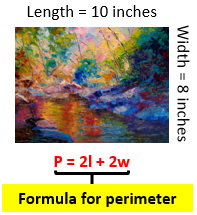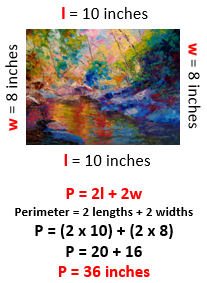# Math Formulas: Lesson for Kids

Instructor: Heather Jenkins

Heather has a bachelor's degree in elementary education and a master's degree in special education. She was a public school teacher and administrator for 11 years.

Wouldn't it be great if someone created a quick way to remember how to solve common math problems? Well, it's already happened! Let's get ready to learn about math formulas and how they help us solve math problems.

## Solve the Mystery

Have you ever read or heard about the famous detective Sherlock Holmes? In his stories, Sherlock is always working to solve an impossible mystery. However, by piecing together clue after clue, he solves the case! When we use formulas in math, we are kind of like Sherlock Holmes working step by step to solve a case.

A math formula is a set of letters and/or numbers that tells you what information you need and what you need to do to find a solution to a specific type of math problem. Math formulas contain variables, which are letters or symbols that stand for a number which is unknown. These variables help you identify what information you need to know to solve the problem. The formula then shows you what to do with those variables, so that you can get the answer!

## Paint a Picture!

Imagine that you painted a picture for a friend! You now want to make a frame for it. What do you need to know in order to make a frame that fits your painting? Well, you would need to know the perimeter, or distance around the picture. To find the perimeter of this picture, you would use the formula for the perimeter of a rectangle: P = 2l + 2w.The variables in the formula, perimeter (p), length (l), and width (w) are like clues letting you know what information you need to solve the problem. The formula shows what steps to take to solve the problem. In this example, the formula shows you that the perimeter is equal to 2 lengths plus 2 widths. So what information do you already know? The picture has a length of 10 inches and a width of 8 inches, right? So, you can use the formula to figure out the perimeter equals 36 inches.Math mystery solved! Take that Sherlock Holmes!

## Example Formulas

Aside from the perimeter of a rectangle, there are a lot of math formulas that people use every single day. Can you think of a formula?

Here are a few examples of formulas that you may run into as you learn more math:

To unlock this lesson you must be a Study.com Member.

### Register to view this lesson

Are you a student or a teacher?

### Unlock Your Education

#### See for yourself why 30 million people use Study.com

##### Become a Study.com member and start learning now.
Back
What teachers are saying about Study.com

### Earning College Credit

Did you know… We have over 200 college courses that prepare you to earn credit by exam that is accepted by over 1,500 colleges and universities. You can test out of the first two years of college and save thousands off your degree. Anyone can earn credit-by-exam regardless of age or education level.